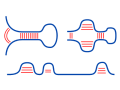Freiburg RNA Tools
Teaching - counting# Teaching - counting : number of structures source at github@BackofenLab/RNA-Playground

The number of nested secondary structures that can be formed by an RNA sequence grows exponentially with sequence length $n$ (about $\sim 2.3^{n}$, see (Hofacker et al., 1997)).

Here, we use a variant of the algorithm by Michael S. Waterman and Temple F. Smith (1970) to compute the exact number of nested structures a sequence can form. The approach applies dynamic programming, i.e. tabularizes results for subproblems. To this end, it populates a matrix $C$, where an entry $C_{i,j}$ provides the exact number of admissible structures for the subsequence from position $i$ to $j$. The overall number of admissible structures for the sequences can be found in the upper right corner $C_{1,n}$, where $n$ denotes the sequence's length and Watson-Crick as well as GU base pairs are considered complementary.RNA sequence $S$:
Minimal loop length $l$:
Recursion for counting the number of possible structures: